# Dedekind ring

An associative-commutative ringwith a unit having no divisors of zero (i.e. a commutative integral domain) in which each proper ideal can be represented as a product of prime ideals (an idealofis said to be prime if the quotient ring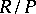does not contain divisors of zero). This name was given to such rings in honour of R. Dedekind, who was one of the first to study such rings in the 1870s.
Any principal ideal domain is a Dedekind ring. Ifis a Dedekind ring andis a finite algebraic extension of its quotient field, the integral closure ofin(i.e. the totality of elements ofwhich are roots of equations of the type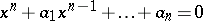,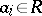) will again be a Dedekind ring. In particular, one has that the rings of algebraic integers and maximal orders in algebraic number fields are Dedekind, i.e. the integral closures of rings of integers in finite algebraic extensions of the field of rational numbers.
In a Dedekind ringeach proper ideal can be uniquely represented as a product of prime ideals. This theorem arose in the problem of decomposition of elements into prime factors in maximal orders in algebraic number fields. Such a decomposition is, as a rule, not unique.
A ringis a Dedekind ring if and only if the semi-group of fractional ideals (cf. Fractional ideal) of this ring is a group. Each fractional ideal of a Dedekind ringcan be uniquely represented as the product of (positive or negative) powers of prime ideals of. A Dedekind ring can be characterized as follows: A commutative integral domainis a Dedekind ring if and only ifis a Noetherian ring, if each proper prime ideal of the ringis maximal and ifis integrally closed, i.e. coincides with its integral closure in its field of fractions. In other words, a Dedekind ring is a Noetherian normal ring of Krull dimension one. The so-called Chinese remainder theorem is valid for a Dedekind ring: For a given finite selection of ideals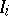and elements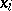of,, the system of congruences(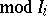) has a solution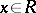if and only if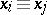() for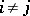. A Dedekind ringmay also be characterized as a Krull ring of dimension one. Each Dedekind ring is a regular commutative ring and all its localizations by maximal ideals form a discretely-normed ring. The semi-group of non-zero ideals of a Dedekind ringis isomorphic to the semi-groupof divisors (cf. Divisor) of this ring.